Do you like the article?
Share it with others -

Use new possibilities of MetaTrader 5

# Free-of-Holes Charts

20 June 2006, 12:392
6 746

### 1. Motivation

In MT 4, only those bars are drawn, within which at least one price change took place. If no price change occurred within a minute, a one-bar gap will occur in the chart with one-minute period.

The developers have deliberately chosen this way of charts drawing since the most traders that use their product prefer charts, which contain only existing prices. Nevertheless, there are users who like continuous charts. They prefer the bar to be drawn if even its open price is equal to the close price of the preceding bar. Thus, there will be no gaps in the time scale of the chart, and 100 bars will always correspond with 100 minutes in the one-minute chart. These data can be different in the current realization. For example, 100 minutes can "fit" in 98 bars if there were 2 minutes among them when no quotes had income.

Fortunately, there are all necessary tools in MQL4 that can help to draw such charts independently.

### 2. Realization

First, let us split the task into two stages:

• history data processing

• latest bar updating

At the first stage, we create a new history file with prefix "ALL" before the symbol name ("ALL" means "all bars" here) and write the history with the added bars into it.

A similar problem is solved in the "period_converter" script that is delivered with МТ 4 Client Terminal. The script generates a chart with a non-standard period. We will use this example to learn how to work with the history file.

Before creation of a program, we have to decide what form will it have: Will it be a script, an indicator, or an expert? Indicators are used to display the contents of an array. We don't need it here. As to scripts and experts, the only difference between them is that scripts are deleted from the chart immediately after their operation has been completed. This suits us at this stage, so this will be script that we are going to produce now.

This is what we get as a result (AllMinutes_Step1.mq4):

```#property show_inputs

//---- Enable/disable drawing bars on holidays
//---- If == true, holidays will remain unfilled
//---- If == false, holidays will be filled out with bars O=H=L=C
extern bool  SkipWeekEnd=true;

int start()
{
int HistoryHandle=-1,pre_time,now_time,_PeriodSec;
double  now_close,now_open,now_low,now_high,now_volume,pre_close;

int    temp;

//---- remember the chart symbol and period
string _Symbol=Symbol();
int _Period= Period();
_PeriodSec = _Period * 60;

//---- open file, in which we will write the history
string file_name=StringConcatenate("ALL",_Symbol,_Period,".hst");
HistoryHandle=FileOpenHistory(file_name,FILE_BIN|FILE_WRITE);
if(HistoryHandle<0)
{
_GetLastError=GetLastError();
Alert("FileOpenHistory( \"",file_name,"\", FILE_BIN | FILE_WRITE )"," - Error #",_GetLastError);
return(-1);
}

FileWriteInteger(HistoryHandle,400,LONG_VALUE);
FileWriteString(HistoryHandle,"ALL"+_Symbol,12);
FileWriteInteger(HistoryHandle,_Period,LONG_VALUE);
FileWriteInteger(HistoryHandle,Digits,LONG_VALUE);
FileWriteInteger(HistoryHandle,0,LONG_VALUE);       //timesign
FileWriteInteger(HistoryHandle,0,LONG_VALUE);       //last_sync
FileWriteArray(HistoryHandle,temp,0,13);

//+-----------------------------------------------------------------+
//| Process the history
//+-----------------------------------------------------------------+
int bars=Bars;
pre_time=Time[bars-1];
for(int i=bars-1; i>=0; i--)
{
//---- Remember the bar parameters
now_open=Open[i];
now_high=High[i];
now_low=Low[i];
now_close=Close[i];
now_volume=Volume[i];
now_time=Time[i]/_PeriodSec;
now_time*=_PeriodSec;

//---- if there are skipped bars,
while(now_time>pre_time+_PeriodSec)
{
pre_time+=_PeriodSec;
pre_time    /= _PeriodSec;
pre_time    *= _PeriodSec;

//---- if it is not the weekend,
if(SkipWeekEnd)
{
if(TimeDayOfWeek(pre_time)<=0 ||
TimeDayOfWeek(pre_time)>5)
{
continue;
}
if(TimeDayOfWeek(pre_time)==5)
{
if( TimeHour(pre_time) == 23 ||
TimeHour(pre_time + _PeriodSec) == 23 )
{
continue;
}
}
}

//---- write the skipped bar into the file
FileWriteInteger(HistoryHandle,pre_time,LONG_VALUE);
FileWriteDouble(HistoryHandle,pre_close,DOUBLE_VALUE);
FileWriteDouble(HistoryHandle,pre_close,DOUBLE_VALUE);
FileWriteDouble(HistoryHandle,pre_close,DOUBLE_VALUE);
FileWriteDouble(HistoryHandle,pre_close,DOUBLE_VALUE);
FileWriteDouble(HistoryHandle,0,DOUBLE_VALUE);
FileFlush(HistoryHandle);
}

//---- write the new bar into the file
FileWriteInteger(HistoryHandle,now_time,LONG_VALUE);
FileWriteDouble(HistoryHandle,now_open,DOUBLE_VALUE);
FileWriteDouble(HistoryHandle,now_low,DOUBLE_VALUE);
FileWriteDouble(HistoryHandle,now_high,DOUBLE_VALUE);
FileWriteDouble(HistoryHandle,now_close,DOUBLE_VALUE);
FileWriteDouble(HistoryHandle,now_volume,DOUBLE_VALUE);
FileFlush(HistoryHandle);
cnt_copy++;

//---- remember the time value and the close price
//---- of the recorded bar
pre_close=now_close;
pre_time=now_time/_PeriodSec;
pre_time*=_PeriodSec;
}

//---- close the file
FileClose(HistoryHandle);

//---- display statistics
Print("< - - - ",_Symbol,_Period,": there were ",cnt_copy,
Print("< - - - To view the results, open the chart \"ALL",
_Symbol,_Period,"\" - - - >");
return(0);
}```

It is recommended to pay attention to the SkipWeekEnd variable. If its value is false, the weekends will be filled with bars O=H=L=C (en dashes).

Let us check how our script works by simple attaching it to the one-minute GBPUSD chart: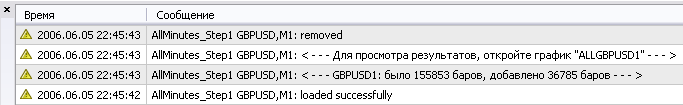Now, let us open the ALLGBPUSD1 chart in offline mode and compare it to the initial chart: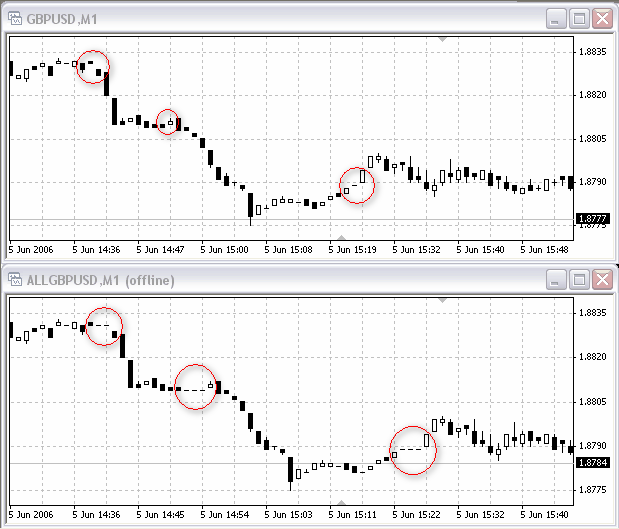As you can see, some skipped minutes were added into the chart. They are circled in red. This is what we wanted to achieve, isn't it?

As we have a chart with the holes filled now, we can update it. The new quotes will be displayed in it, but the new holes will remain unfilled again.

The "period_converter" script can be used as an example again. It can solve the problem of the charts updating, as well. We will make only one change: Add the block of filling the skipped bars.  Since the chart must be updated at every tick, let us transfer our code into the expert. It will be launched every time when a new quote incomes. Let us place the code from the first part into the init() function since this part must be executed only once, and the entire new part of the code will be placed in the start() function since it will be used every tick. Besides, the file closing will go to the deinit(), it is the right place for it.

Thus, the expert code (AllMinutes_Step2.mq4) is as follows:

```#include <WinUser32.mqh>

//---- Enable/disable drawing of bars on holidays
//---- If it is == true, the holidays will remain unfilled
//---- If it is == false, the holidays will be filled with the O=H=L=C bars
extern bool  SkipWeekEnd=true;

int  HistoryHandle=-1,hwnd=0,last_fpos=0,pre_time,now_time;
int  _Period,_PeriodSec;
double  now_close,now_open,now_low,now_high,now_volume;
double  pre_close,pre_open,pre_low,pre_high,pre_volume;
string  _Symbol;

int init()
{
int    temp;

//---- remember the chart symbol and period
_Symbol=Symbol();
_Period=Period();
_PeriodSec=_Period*60;
hwnd=0;

//---- open the file to write the history in
string file_name=StringConcatenate("ALL",_Symbol,_Period,".hst");
HistoryHandle=FileOpenHistory(file_name,FILE_BIN|FILE_WRITE);
if(HistoryHandle<0)
{
_GetLastError=GetLastError();
Alert("FileOpenHistory( \"",file_name,"\", FILE_BIN | FILE_WRITE )"," - Error #",_GetLastError);
return(-1);
}

FileWriteInteger(HistoryHandle,400,LONG_VALUE);
FileWriteString(HistoryHandle,StringConcatenate("ALL",_Symbol),
12);
FileWriteInteger(HistoryHandle,_Period,LONG_VALUE);
FileWriteInteger(HistoryHandle,Digits,LONG_VALUE);
FileWriteInteger(HistoryHandle,0,LONG_VALUE);       //timesign
FileWriteInteger(HistoryHandle,0,LONG_VALUE);       //last_sync
FileWriteArray(HistoryHandle,temp,0,13);

//+-----------------------------------------------------------------+
//| Process the history
//+-----------------------------------------------------------------+
int bars=Bars;
pre_time=Time[bars-1];
for(int i=bars-1; i>=1; i--)
{
//---- Remember the bar parameters
now_open=Open[i];
now_high=High[i];
now_low=Low[i];
now_close=Close[i];
now_volume=Volume[i];
now_time=Time[i]/_PeriodSec;
now_time*=_PeriodSec;

//---- if there are skipped bars,
while(now_time>pre_time+_PeriodSec)
{
pre_time+=_PeriodSec;
pre_time    /= _PeriodSec;
pre_time    *= _PeriodSec;

//---- if it isn't a weekend,
if(SkipWeekEnd)
{
if(TimeDayOfWeek(pre_time)<=0 || TimeDayOfWeek(pre_time)>5)
{
continue;
}
if(TimeDayOfWeek(pre_time)==5)
{
if( TimeHour(pre_time) == 23 ||
TimeHour(pre_time + _PeriodSec) == 23 )
{
continue;
}
}
}

//---- write the obtained bar into the file
FileWriteInteger(HistoryHandle,pre_time,LONG_VALUE);
FileWriteDouble(HistoryHandle,pre_close,DOUBLE_VALUE);
FileWriteDouble(HistoryHandle,pre_close,DOUBLE_VALUE);
FileWriteDouble(HistoryHandle,pre_close,DOUBLE_VALUE);
FileWriteDouble(HistoryHandle,pre_close,DOUBLE_VALUE);
FileWriteDouble(HistoryHandle,0,DOUBLE_VALUE);
FileFlush(HistoryHandle);
}

//---- write the new bar into the file
FileWriteInteger(HistoryHandle,now_time,LONG_VALUE);
FileWriteDouble(HistoryHandle,now_open,DOUBLE_VALUE);
FileWriteDouble(HistoryHandle,now_low,DOUBLE_VALUE);
FileWriteDouble(HistoryHandle,now_high,DOUBLE_VALUE);
FileWriteDouble(HistoryHandle,now_close,DOUBLE_VALUE);
FileWriteDouble(HistoryHandle,now_volume,DOUBLE_VALUE);
FileFlush(HistoryHandle);
cnt_copy++;

//---- remember the time value and the close price of the recorded
//---- bar
pre_close=now_close;
pre_time=now_time/_PeriodSec;
pre_time*=_PeriodSec;
}

last_fpos=FileTell(HistoryHandle);

//---- display statistics
Print("< - - - ",_Symbol,_Period,": there were ",cnt_copy," bars, added ",cnt_add," bars - - - >");
Print("< - - - To view the results, open the chart \"ALL",
_Symbol,_Period,"\" - - - >");

//---- call the start function for the 0th bar to be drawn immediately
start();

return(0);
}
//----
int start()
{
//+---------------------------------------------------------------+
//| Process the incoming ticks
//+---------------------------------------------------------------+

//---- place the "cursor" before the latest bar
//---- (this must be done at all launches except for the first one)
FileSeek(HistoryHandle,last_fpos,SEEK_SET);

//---- Remember the bar parameters
now_open=Open;
now_high=High;
now_low=Low;
now_close=Close;
now_volume=Volume;
now_time=Time/_PeriodSec;
now_time*=_PeriodSec;

//---- if the bar has been formed,
if(now_time>=pre_time+_PeriodSec)
{
//---- write the newly formed bar
FileWriteInteger(HistoryHandle,pre_time,LONG_VALUE);
FileWriteDouble(HistoryHandle,pre_open,DOUBLE_VALUE);
FileWriteDouble(HistoryHandle,pre_low,DOUBLE_VALUE);
FileWriteDouble(HistoryHandle,pre_high,DOUBLE_VALUE);
FileWriteDouble(HistoryHandle,pre_close,DOUBLE_VALUE);
FileWriteDouble(HistoryHandle,pre_volume,DOUBLE_VALUE);
FileFlush(HistoryHandle);

//---- remember the position in the file, before writing of the 0th bar
last_fpos=FileTell(HistoryHandle);
}

//---- if the skipped bars have appeared,
while(now_time>pre_time+_PeriodSec)
{
pre_time+=_PeriodSec;
pre_time /= _PeriodSec;
pre_time *= _PeriodSec;

//---- if this is not weekend,
if(SkipWeekEnd)
{
if(TimeDayOfWeek(pre_time)<=0 ||
TimeDayOfWeek(pre_time)>5)
{
continue;
}
if(TimeDayOfWeek(pre_time)==5)
{
if(TimeHour(pre_time)==23 ||
TimeHour(pre_time+_PeriodSec)==23)
{
continue;
}
}
}

//---- write the skipped bar into the file
FileWriteInteger(HistoryHandle,pre_time,LONG_VALUE);
FileWriteDouble(HistoryHandle,pre_close,DOUBLE_VALUE);
FileWriteDouble(HistoryHandle,pre_close,DOUBLE_VALUE);
FileWriteDouble(HistoryHandle,pre_close,DOUBLE_VALUE);
FileWriteDouble(HistoryHandle,pre_close,DOUBLE_VALUE);
FileWriteDouble(HistoryHandle,0,DOUBLE_VALUE);
FileFlush(HistoryHandle);

//---- remember the position in the file, before writing of the 0th bar
last_fpos=FileTell(HistoryHandle);
}

//---- write the current bar
FileWriteInteger(HistoryHandle,now_time,LONG_VALUE);
FileWriteDouble(HistoryHandle,now_open,DOUBLE_VALUE);
FileWriteDouble(HistoryHandle,now_low,DOUBLE_VALUE);
FileWriteDouble(HistoryHandle,now_high,DOUBLE_VALUE);
FileWriteDouble(HistoryHandle,now_close,DOUBLE_VALUE);
FileWriteDouble(HistoryHandle,now_volume,DOUBLE_VALUE);
FileFlush(HistoryHandle);

//---- remember the parameters of the recorded bar
pre_open=now_open;
pre_high=now_high;
pre_low=now_low;
pre_close=now_close;
pre_volume=now_volume;
pre_time=now_time/_PeriodSec;
pre_time*=_PeriodSec;

//---- find the window the new quotes to be "sent" to
if(hwnd==0)
{
hwnd = WindowHandle( StringConcatenate( "ALL", _Symbol ), _Period );
if( hwnd != 0 )
{
Print("< - - - Chart ",
"ALL"+_Symbol,_Period," has been found! - - - >");
}
}
//---- and, if successfully found, update it
if(hwnd!=0)
{
PostMessageA(hwnd,WM_COMMAND,33324,0);
}
}
//----
int deinit()
{
if(HistoryHandle>=0)
{
//---- close the file
FileClose(HistoryHandle);
HistoryHandle=-1;
}
return(0);
}```

One reservation must be made first: The updating of the chart is rather processor-intensive, since the terminal loads all bars written in the file. If there are many bars in the file, the terminal can  become much slower. This mostly depends on the perfomance of PC where the МТ 4 Client Terminal is installed. In any case, resources are not inexhaustible. We will solve this problem in a simple way: Just reduce the amount of bars displayed in the chart to 10,000 ("Tools" – "Options" – "Charts", the "Max bars in chart" parameter). Now let us restart the terminal and attach the expert: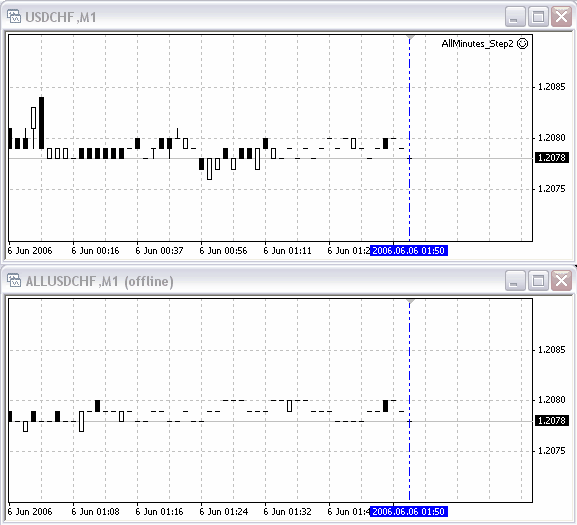The expert has immediately "mended" the history and is waiting until the new ticks appear. After 2 minutes, the same charts appeared as follows: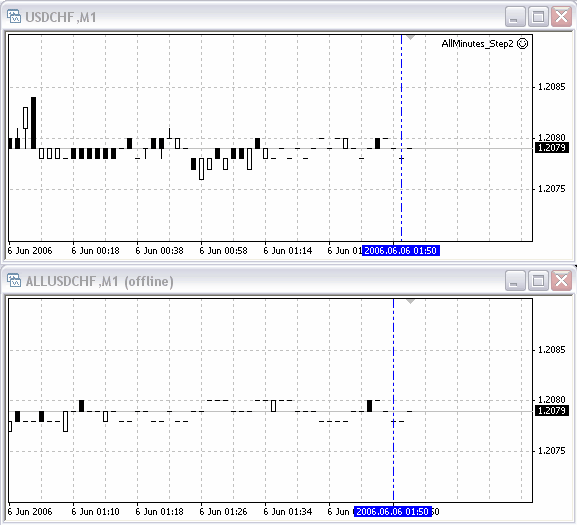As you can see, a one-minute bar was added in the upper chart while the skipped bar was added into the lower chart.

This means we have effected the desired result!

### 3.Scaling

One chart is ok, of course, but what should we do if there is a need to open 10 charts without skipped bars? Opening of an additional, "temporary" chart for every normal chart would not be the best solution. The extra resources will be spent and, respectively, the working will be less comfortable.

Let us create an expert that would process any amount of charts. This will be a suitable and saving solution.

Thus, we will have to modify our code to make it working with several charts simultaneously:

• add an external variable that would help to change the list of charts,
• replace all variables with arrays having the amount of elements equal to the amount of charts to be processed,
• place the entire code into a loop where these charts will be searched, and
• put the updating block into the infinite loop, having become independent on the quotes income in such a way. If different symbols are listed, their updating time can be different, as well.

This is what we should have drawn as a result (AllMinutes.mq4):

```#include <WinUser32.mqh>

//---- The list of charts to be processed, separated by commas (",")
extern string    ChartList="EURUSD1,GBPUSD1";
//---- Enable/disable to draw bars at weekends
//---- If it is == true, the weekends will remain unfilled
//---- If it is == false, the weekends will be filled with the bars of O=H=L=C
extern bool     SkipWeekEnd=true;
//---- Frequency of the charts updating, in milliseconds
//---- the higher the value is, the less resources the expert
//---- will use.
extern int   RefreshLuft=1000;

int init()
{
start();
return(0);
}

int start()
{
int   Charts=0,pos=0,curchar=0,len=StringLen(ChartList);
string    cur_symbol="",cur_period="",file_name="";

string    _Symbol; int _Period,_PeriodSec[],_Bars[];
int HistoryHandle[],hwnd[],last_fpos[],pre_time[],now_time[];
double   now_close[],now_open[],now_low[],now_high[],now_volume[];
double   pre_close[],pre_open[],pre_low[],pre_high[],pre_volume[];

//---- count the amount of charts to be processed
while(pos<=len)
{
curchar=StringGetChar(ChartList,pos);
if(curchar>47 && curchar<58)
{
cur_period=cur_period+CharToStr(curchar);
}
else
{
if(curchar==',' || pos==len)
{
MarketInfo(cur_symbol,MODE_BID);
if(GetLastError()==4106)
{
return(-1);
}
if(iClose(cur_symbol,StrToInteger(cur_period),0)<=0)
{
return(-1);
}

_Symbol[Charts]=cur_symbol;
_Period[Charts]=StrToInteger(cur_period);
cur_symbol=""; cur_period="";

Charts++;
}
else
{
cur_symbol=cur_symbol+CharToStr(curchar);
}
}
pos++;
}
Print("< - - - Found ",Charts," correct charts. - - - >");
ArrayResize(_Symbol,Charts);
ArrayResize(_Period,Charts);
ArrayResize(HistoryHandle,Charts);
ArrayResize(hwnd,Charts);
ArrayResize(last_fpos,Charts);
ArrayResize(pre_time,Charts);
ArrayResize(now_time,Charts);
ArrayResize(now_close,Charts);
ArrayResize(now_open,Charts);
ArrayResize(now_low,Charts);
ArrayResize(now_high,Charts);
ArrayResize(now_volume,Charts);
ArrayResize(pre_close,Charts);
ArrayResize(pre_open,Charts);
ArrayResize(pre_low,Charts);
ArrayResize(pre_high,Charts);
ArrayResize(pre_volume,Charts);
ArrayResize(_PeriodSec,Charts);
ArrayResize(_Bars,Charts);

for(int curChart=0; curChart<Charts; curChart++)
{
_PeriodSec[curChart]=_Period[curChart] *60;

//---- open the file the history to be written in
file_name=StringConcatenate("ALL",_Symbol[curChart],
_Period[curChart],".hst");
HistoryHandle[curChart]=FileOpenHistory(file_name,
FILE_BIN|FILE_WRITE);
if(HistoryHandle[curChart]<0)
{
_GetLastError=GetLastError();
Alert("FileOpenHistory( \"",file_name,"\", FILE_BIN | FILE_WRITE )"," - Error #",_GetLastError);
continue;
}

FileWriteInteger(HistoryHandle[curChart],400,LONG_VALUE);
FileWriteString(HistoryHandle[curChart],
FileWriteString(HistoryHandle[curChart],
StringConcatenate("ALL",
_Symbol[curChart]),12);
FileWriteInteger(HistoryHandle[curChart],_Period[curChart],
LONG_VALUE);
FileWriteInteger(HistoryHandle[curChart],
MarketInfo(_Symbol[curChart],
MODE_DIGITS),LONG_VALUE);
FileWriteInteger(HistoryHandle[curChart],0,
LONG_VALUE);       //timesign
FileWriteInteger(HistoryHandle[curChart],0,
LONG_VALUE);       //last_sync
FileWriteArray(HistoryHandle[curChart],temp,0,13);

//+-----------------------------------------------------------+
//| Process the history
//+-----------------------------------------------------------+
_Bars[curChart]=iBars(_Symbol[curChart],_Period[curChart]);
pre_time[curChart]=iTime(_Symbol[curChart],
_Period[curChart],_Bars[curChart]-1);
for(int i=_Bars[curChart]-1; i>=1; i--)
{
//---- Remember the bar parameters
now_open[curChart]=iOpen(_Symbol[curChart],
_Period[curChart],i);
now_high[curChart]=iHigh(_Symbol[curChart],
_Period[curChart],i);
now_low[curChart]=iLow(_Symbol[curChart],
_Period[curChart],i);
now_close[curChart]=iClose(_Symbol[curChart],
_Period[curChart],i);
now_volume[curChart]=iVolume(_Symbol[curChart],
_Period[curChart],i);
now_time[curChart]=iTime(_Symbol[curChart],
_Period[curChart],i)
/_PeriodSec[curChart];
now_time[curChart]*=_PeriodSec[curChart];

//---- if there are skipped bars,
while(now_time[curChart]>pre_time[curChart]+
_PeriodSec[curChart])
{
pre_time[curChart]+=_PeriodSec[curChart];
pre_time[curChart] /= _PeriodSec[curChart];
pre_time[curChart] *= _PeriodSec[curChart];

//---- if this is not the weekend,
if(SkipWeekEnd)
{
if(TimeDayOfWeek(pre_time[curChart])<=0 ||
TimeDayOfWeek(pre_time[curChart])>5)
{
continue;
}
if(TimeDayOfWeek(pre_time[curChart])==5)
{
if(TimeHour(pre_time[curChart])==23 ||
TimeHour(pre_time[curChart]+_PeriodSec[curChart])==23)
{
continue;
}
}
}

//---- write the skipped bar into the file
FileWriteInteger(HistoryHandle[curChart],pre_time[curChart],
LONG_VALUE);
FileWriteDouble(HistoryHandle[curChart],
pre_close[curChart],DOUBLE_VALUE);
FileWriteDouble(HistoryHandle[curChart],
pre_close[curChart],DOUBLE_VALUE);
FileWriteDouble(HistoryHandle[curChart],
pre_close[curChart],DOUBLE_VALUE);
FileWriteDouble(HistoryHandle[curChart],
pre_close[curChart],DOUBLE_VALUE);
FileWriteDouble(HistoryHandle[curChart],0,
DOUBLE_VALUE);
FileFlush(HistoryHandle[curChart]);
}

//---- write the new bar into the file
FileWriteInteger(HistoryHandle[curChart],now_time[curChart],
LONG_VALUE);
FileWriteDouble(HistoryHandle[curChart],
now_open[curChart],DOUBLE_VALUE);
FileWriteDouble(HistoryHandle[curChart],
now_low[curChart],DOUBLE_VALUE);
FileWriteDouble(HistoryHandle[curChart],
now_high[curChart],DOUBLE_VALUE);
FileWriteDouble(HistoryHandle[curChart],
now_close[curChart],DOUBLE_VALUE);
FileWriteDouble(HistoryHandle[curChart],
now_volume[curChart],DOUBLE_VALUE);
FileFlush(HistoryHandle[curChart]);
cnt_copy++;

//---- remember the time value and the close price
//---- of the recorded bar
pre_close[curChart]=now_close[curChart];
pre_time[curChart]=now_time[curChart]/
_PeriodSec[curChart];
pre_time[curChart]*=_PeriodSec[curChart];
}

last_fpos[curChart]=FileTell(HistoryHandle[curChart]);

//---- display statistics
Print("< - - - ",_Symbol[curChart],_Period[curChart],": there were ",
Print("< - - - To view the results, open chart \"ALL",
_Symbol[curChart],_Period[curChart],"\" - - - >");

}

//+---------------------------------------------------------------+
//| Process the incoming ticks
//+---------------------------------------------------------------+
while(!IsStopped())
{
RefreshRates();
for(curChart=0; curChart<Charts; curChart++)
{
//---- put the "cursor" before the latest bar
//---- (this must be done at all launches except for the first one)
FileSeek(HistoryHandle[curChart],last_fpos[curChart],
SEEK_SET);

//---- Remember the bar parameters
now_open[curChart]=iOpen(_Symbol[curChart],
_Period[curChart],0);
now_high[curChart]=iHigh(_Symbol[curChart],
_Period[curChart],0);
now_low[curChart]=iLow(_Symbol[curChart],
_Period[curChart],0);
now_close[curChart]=iClose(_Symbol[curChart],
_Period[curChart],0);
now_volume[curChart]=iVolume(_Symbol[curChart],
_Period[curChart],0);
now_time[curChart]=iTime(_Symbol[curChart],
_Period[curChart],0)
/_PeriodSec[curChart];
now_time[curChart]*=_PeriodSec[curChart];

//---- if a bar has been formed,
if(now_time[curChart]>=pre_time[curChart]+
_PeriodSec[curChart])
{
//---- write the formed bar
FileWriteInteger(HistoryHandle[curChart],pre_time[curChart],
LONG_VALUE);
FileWriteDouble(HistoryHandle[curChart],
pre_open[curChart],DOUBLE_VALUE);
FileWriteDouble(HistoryHandle[curChart],
pre_low[curChart],DOUBLE_VALUE);
FileWriteDouble(HistoryHandle[curChart],
pre_high[curChart],DOUBLE_VALUE);
FileWriteDouble(HistoryHandle[curChart],
pre_close[curChart],DOUBLE_VALUE);
FileWriteDouble(HistoryHandle[curChart],
pre_volume[curChart],DOUBLE_VALUE);
FileFlush(HistoryHandle[curChart]);

//---- remember the position in the file before recording the 0th bar
last_fpos[curChart]=FileTell(HistoryHandle[curChart]);
}

//---- if skipped bars appear,
while(now_time[curChart]>pre_time[curChart]+_PeriodSec[curChart])
{
pre_time[curChart] += _PeriodSec[curChart];
pre_time[curChart] /= _PeriodSec[curChart];
pre_time[curChart] *= _PeriodSec[curChart];

//---- if this is not the weekend,
if(SkipWeekEnd)
{
if(TimeDayOfWeek(pre_time[curChart])<=0 ||
TimeDayOfWeek(pre_time[curChart])>5)
{
continue;
}
if(TimeDayOfWeek(pre_time[curChart])==5)
{
if(TimeHour(pre_time[curChart])==23 ||
TimeHour(pre_time[curChart]+_PeriodSec[curChart])==23)
{
continue;

}
}
}

//---- write the skipped bar into the file
FileWriteInteger(HistoryHandle[curChart],pre_time[curChart],
LONG_VALUE);
FileWriteDouble(HistoryHandle[curChart],
pre_close[curChart],DOUBLE_VALUE);
FileWriteDouble(HistoryHandle[curChart],
pre_close[curChart],DOUBLE_VALUE);
FileWriteDouble(HistoryHandle[curChart],
pre_close[curChart],DOUBLE_VALUE);
FileWriteDouble(HistoryHandle[curChart],
pre_close[curChart],DOUBLE_VALUE);
FileWriteDouble(HistoryHandle[curChart],0,
DOUBLE_VALUE);
FileFlush(HistoryHandle[curChart]);

//---- remember the position in the file before to record the 0th bar
last_fpos[curChart]=FileTell(HistoryHandle[curChart]);
}

//---- write the current bar
FileWriteInteger(HistoryHandle[curChart],now_time[curChart],
LONG_VALUE);
FileWriteDouble(HistoryHandle[curChart],
now_open[curChart],DOUBLE_VALUE);
FileWriteDouble(HistoryHandle[curChart],
now_low[curChart],DOUBLE_VALUE);
FileWriteDouble(HistoryHandle[curChart],
now_high[curChart],DOUBLE_VALUE);
FileWriteDouble(HistoryHandle[curChart],
now_close[curChart],DOUBLE_VALUE);
FileWriteDouble(HistoryHandle[curChart],
now_volume[curChart],DOUBLE_VALUE);
FileFlush(HistoryHandle[curChart]);

//---- remember the parameters of the written bar
pre_open[curChart]        = now_open[curChart];
pre_high[curChart]        = now_high[curChart];
pre_low[curChart]=now_low[curChart];
pre_close[curChart]=now_close[curChart];
pre_volume[curChart]=now_volume[curChart];
pre_time[curChart]=now_time[curChart]/
_PeriodSec[curChart];
pre_time[curChart]*=_PeriodSec[curChart];

//---- find the window to "send" the new quotes to
if(hwnd[curChart]==0)
{
hwnd[curChart]=WindowHandle(StringConcatenate("ALL",
_Symbol[curChart]),
_Period[curChart]);
if(hwnd[curChart]!=0)
{
Print("< - - - Chart ","ALL"+_Symbol[curChart],
_Period[curChart]," found! - - - >");
}
}
//---- and, if found, update it
if(hwnd[curChart]!=0)
{
PostMessageA(hwnd[curChart],WM_COMMAND,33324,0);
}
}
Sleep(RefreshLuft);
}

for(curChart=0; curChart<Charts; curChart++)
{
if(HistoryHandle[curChart]>=0)
{
//---- close the file
FileClose(HistoryHandle[curChart]);
HistoryHandle[curChart]=-1;
}
}
return(0);
}```

Now let us launch the expert in the 5-minute EURUSD chart with the ChartList parameter being equal to "EURUSD1,GBPUSD1,EURGBP1", and open all the three charts in offline mode: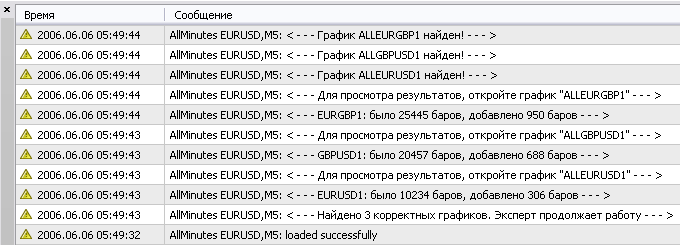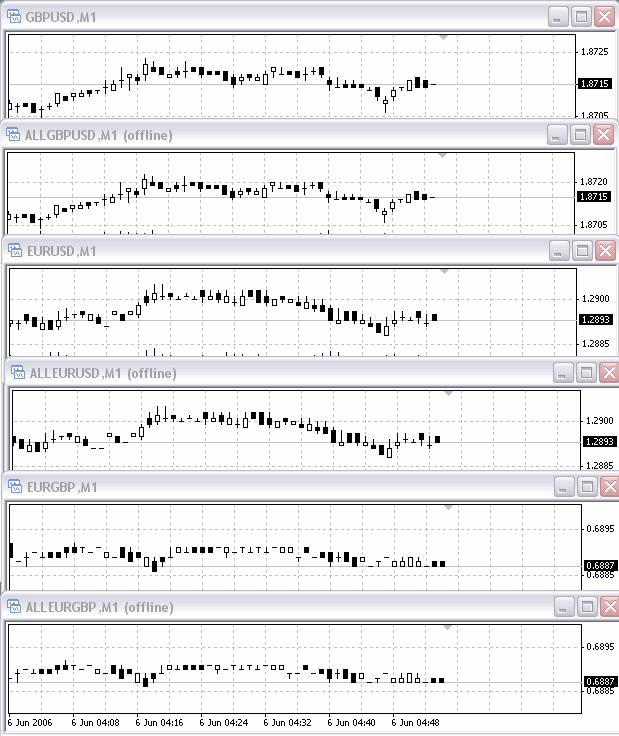Everything seems to be ok: All three charts are updated simultaneously and will be "mended" if some holes appear.

Translated from Russian by MetaQuotes Software Corp.
Original article: https://www.mql5.com/ru/articles/1407

Attached files |
AllMinutes.mq4 (12.72 KB)
Last comments | Go to discussion (2)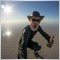| 7 Nov 2017 at 01:14

I don't get it working, someone knows how to fix it, or has another solution?| 7 Nov 2017 at 09:46
Isaak:

I don't get it working, someone knows how to fix it, or has another solution?

Please, use AllMinutes EA version 2.0.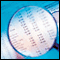One-Minute Data Modelling Quality Rating

One-Minute Data Modelling Quality Rating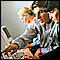Genetic Algorithms: Mathematics

Genetic (evolutionary) algorithms are used for optimization purposes. An example of such purpose can be neuronet learning, i.e., selection of such weight values that allow reaching the minimum error. At this, the genetic algorithm is based on the random search method.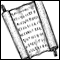Information Storage and View

The article deals with convenient and efficient methods of information storage and viewing. Alternatives to the terminal standard log file and the Comment() function are considered here.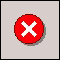Error 146 ("Trade context busy") and How to Deal with It

The article deals with conflict-free trading of several experts on one МТ 4 Client Terminal. It will be useful for those who have basic command of working with the terminal and programming in MQL 4.